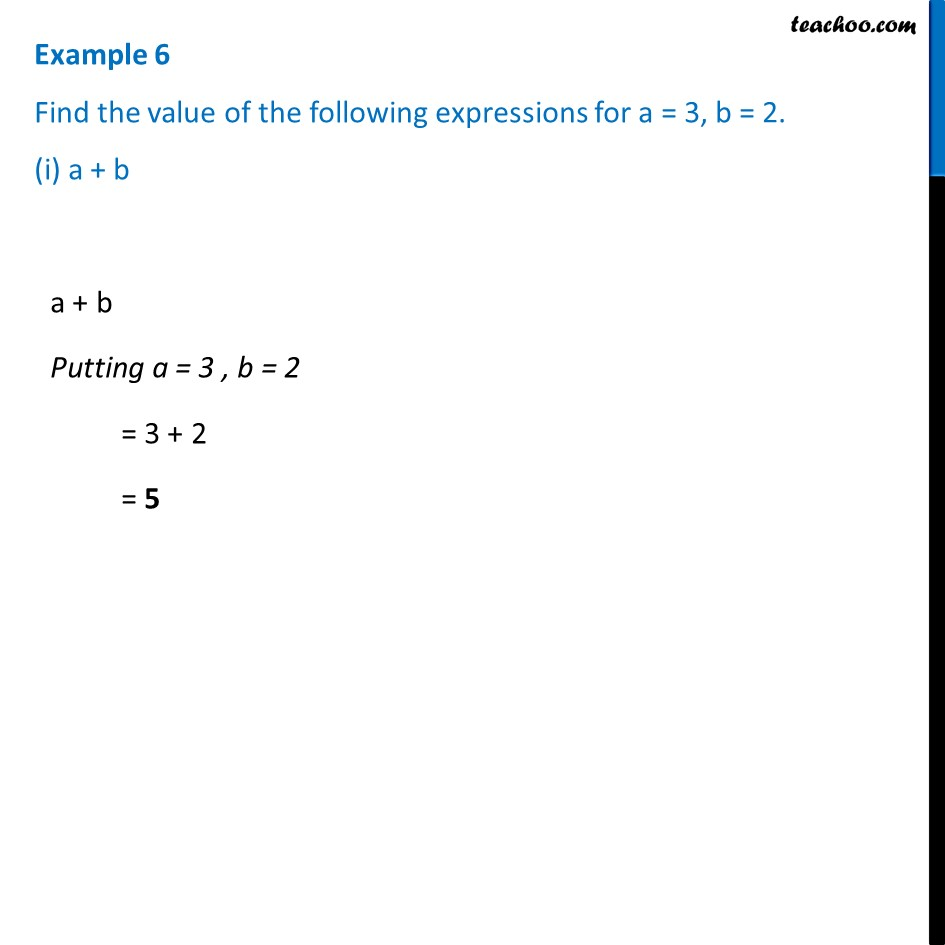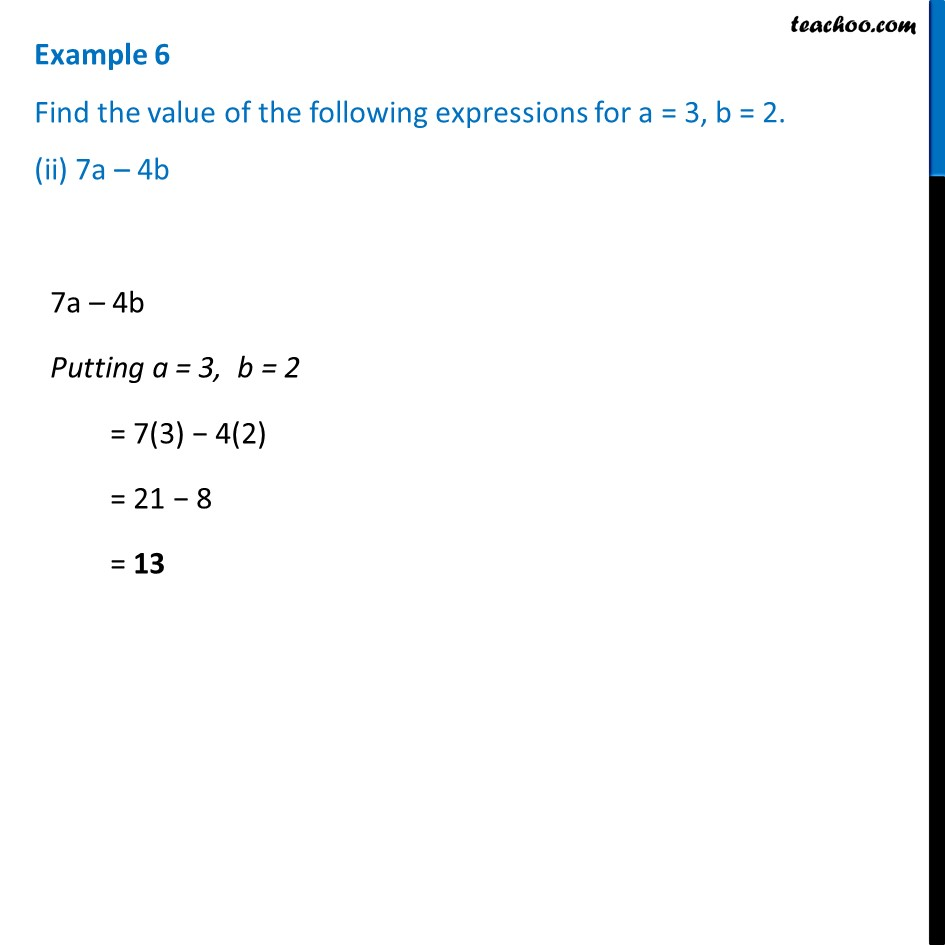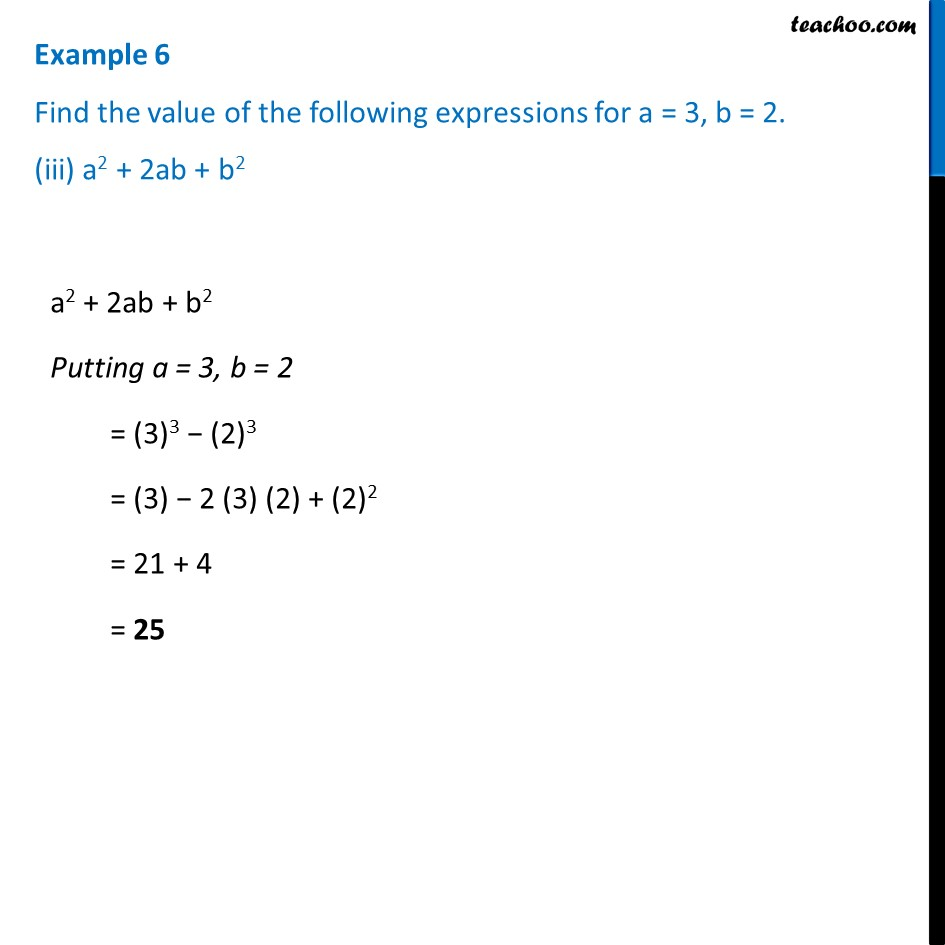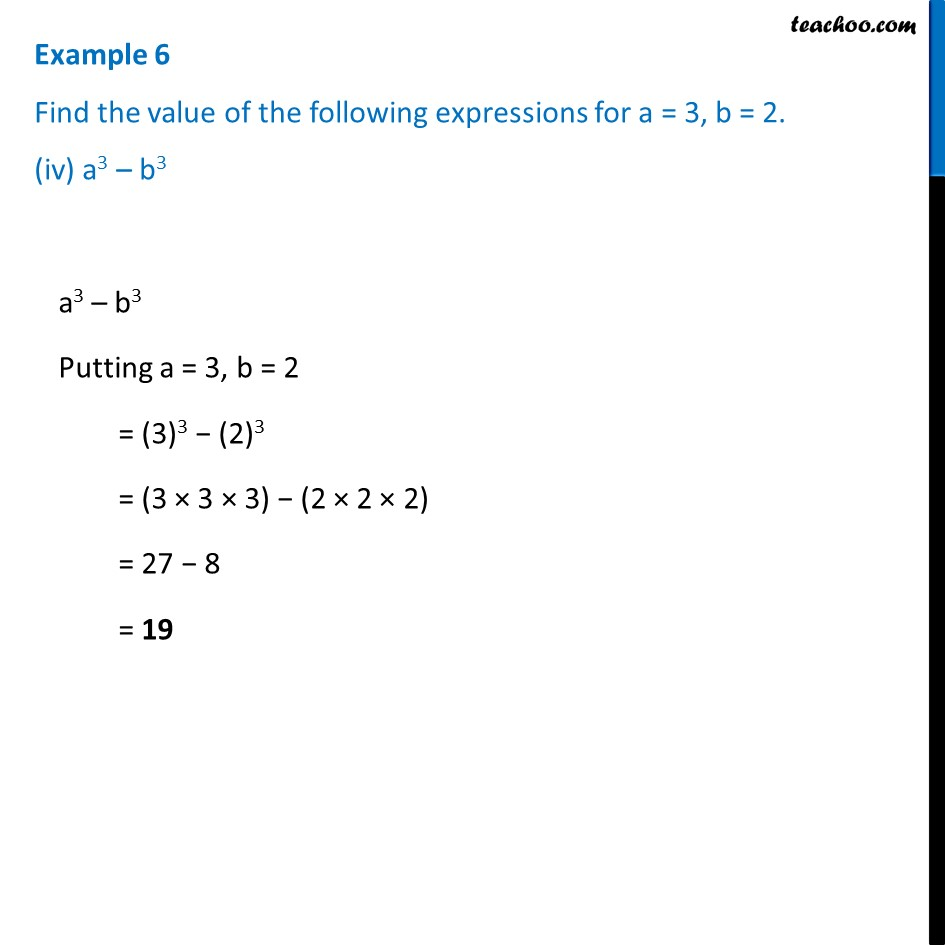Examples

Chapter 10 Class 7 Algebraic Expressions
Serial order wiseLearn in your speed, with individual attention - Teachoo Maths 1-on-1 Class

### Transcript

Example 6 Find the value of the following expressions for a = 3, b = 2. (i) a + b a + b Putting a = 3 , b = 2 = 3 + 2 = 5 Example 6 Find the value of the following expressions for a = 3, b = 2. (ii) 7a – 4b 7a – 4b Putting a = 3, b = 2 = 7(3) − 4(2) = 21 − 8 = 13 Example 6 Find the value of the following expressions for a = 3, b = 2. (iii) a2 + 2ab + b2a2 + 2ab + b2 Putting a = 3, b = 2 = (3)3 − (2)3 = (3) − 2 (3) (2) + (2)2 = 21 + 4 = 25 Example 6 Find the value of the following expressions for a = 3, b = 2. (iv) a3 – b3a3 – b3 Putting a = 3, b = 2 = (3)3 − (2)3 = (3 × 3 × 3) − (2 × 2 × 2) = 27 − 8 = 19